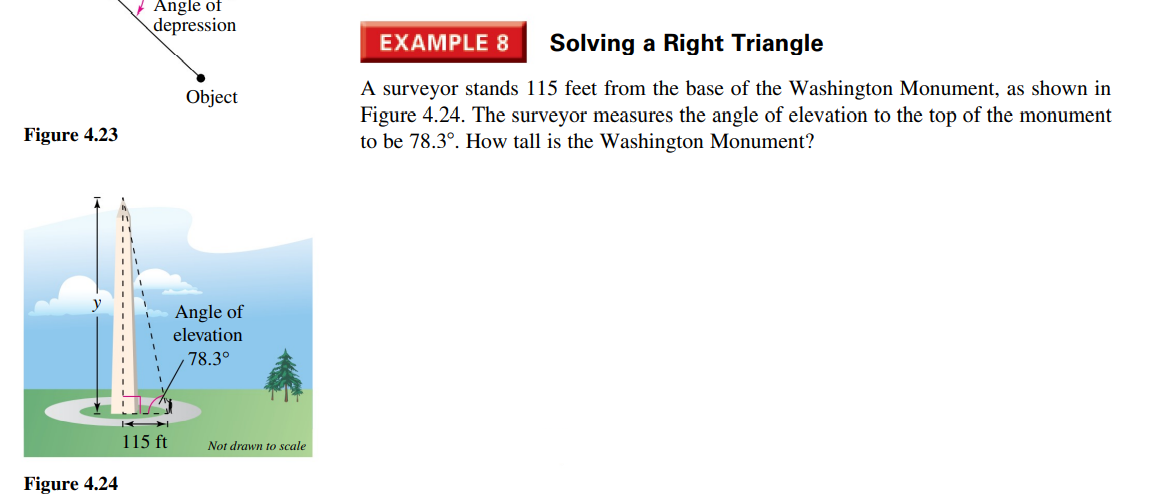### Still have math questions?EXAMPLE $$8$$ Solving a Right Triangle A surveyor stands $$115$$ feet from the base of the Washington Monument, as shown in Figure $$4.24$$ . The surveyor measures the angle of elevation to the top of the monument to be $$78.3 ^ { \circ }$$ . How tall is the Washington Monument?